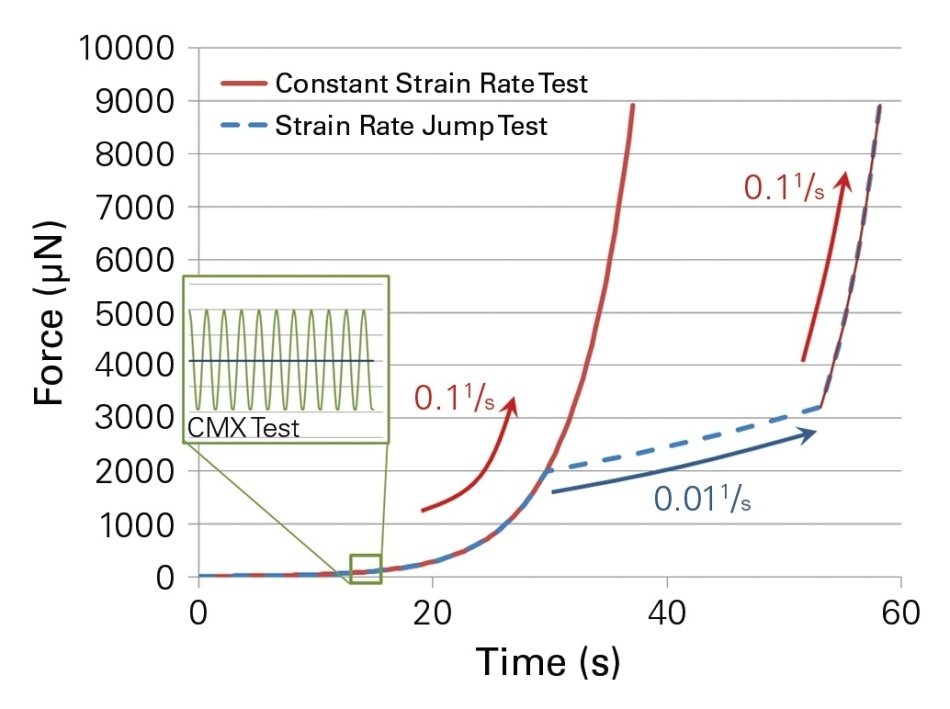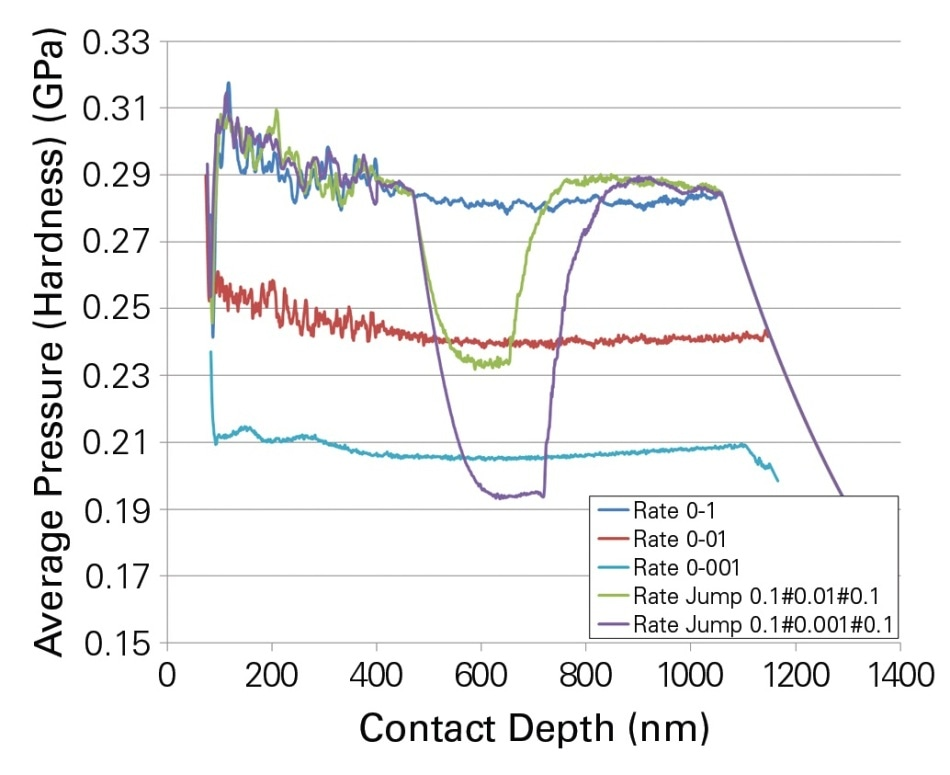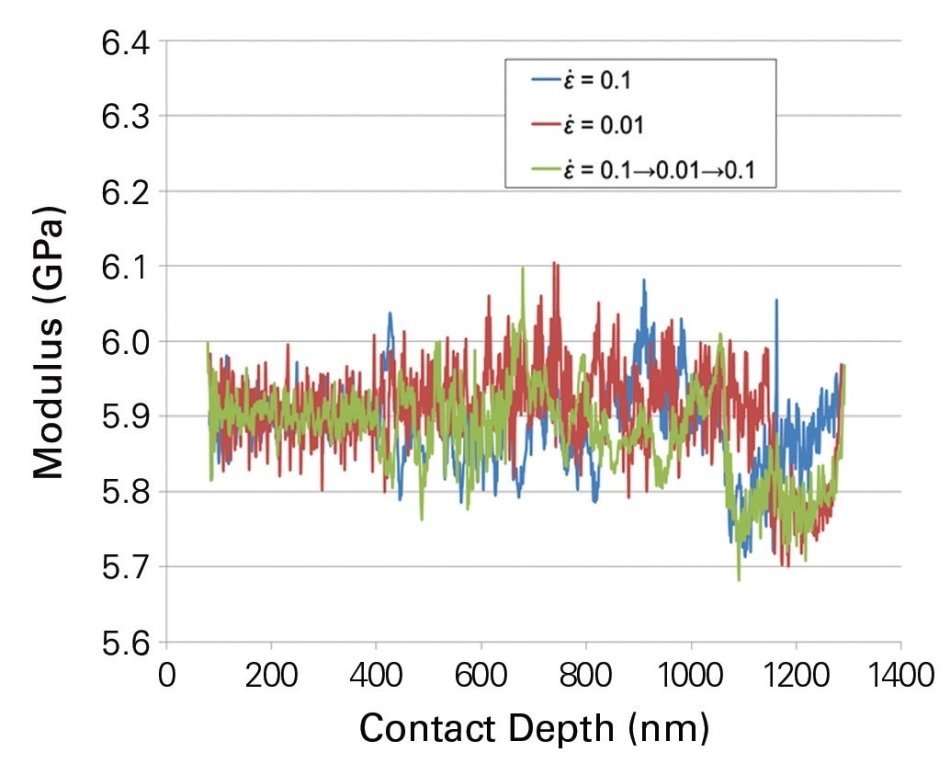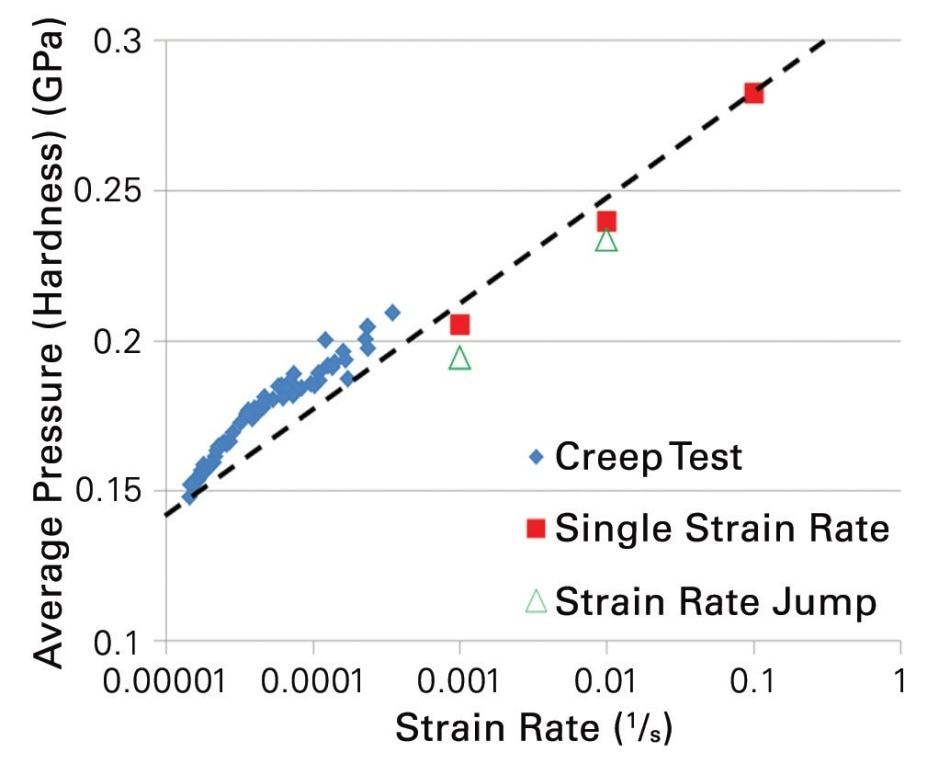# Time-Dependent Deformation Behavior of Polymers

Measurements of hardness or modulus are not usually helpful in studying time-dependent deformation of a material. It is essential to match load functions in order to make sure that measurements are comparable. In a number of cases the materials do not show significant changes in hardness when tested over different time periods, and this means that it is not important to test at a constant strain rate.

Early observations of deformation in a time-based manner, with concept analysis, were obtained in metals with a low melting point which show significant creep behavior. Significant creep is also seen at room temperature with aluminum and copper. Testing at constant strain rates is gaining importance with other metals as well, because with the advanced testing of these materials at raised temperatures, they approach their melting point.

Yet another materials class which requires creep deformation testing is polymers, whose viscoelastic properties change in a time-dependent manner, and this is shown in an experimental setup using Bruker's nanoDMA® III setup or by creep rate analysis if the polymer under study shows low-loss behavior. Creep rates are generally adopted to explore the behavior of materials at low deformation rates, because they evaluate the velocity of deformation of a material in relation to the stress applied.

On the other hand, using a constant strain rate test enables the pressure needed to change the rate of deformation in a controlled manner. Constant strain rate measurements using indentation grids are carried out using a range of strain rates between 1 to 0.001s-1, but for creep experiments it is 0.001 to 0.000001s-1.## Indentation Strain Rate Definition

When tensile testing is done, the strain rate is calculated as the ratio of the velocity of deformation (dl/dt) to the length of the tensile sample. The deformation velocity should not be taken as a function of sample size when an indentation technique is being used. The most obvious scale of length now available is the depth of indenter penetration, hc. The strain rate, dε/dt, is therefore calculated as dhc/dt/hc.

However, the state of applied stress does not exactly correspond to a tensile test. The small hc obtained during the initial testing stages shows that the strain rate can be significantly elevated. If the sample is assumed to have constant hardness, a Berkovich indenter can be used to perform a constant strain rate experiment, fulfilling the strain rate dhc/dt/hc = const., provided the force P changes in a similar manner (dP/dt/P = const.) which in turn mandates that the indentation have an exponential load function.

A change in strain rate affects the indentation in nanocrystalline Al as shown by Meyer et al, in that the level of stress required to attain a certain rate of strain is represented by the hardness in the form of the average pressure under the indenter. This hardness is a function of the alteration in strain rate as specified by the load function. At any strain rate some time is required to reach a steady-state measure of hardness.

In this study, PMMA, which is a glassy polymer, was used at room temperature, comparing constant strain rate experiments with strain rate jump, as well as creep studies when the strain rate was very low.

## Indentation Testing on PMMA

The current indentation experiments were conducted using a PMMA sample which had varying strain rates, namely, 0.1, 0.01, and 0.001s-1as well as with a strain rate jump setup beginning with an initial strain rate of 0.1s-1, changing to 0.01 and 0.001s-1 when the load was 1900 μN.

In addition, a reference creep test was performed over 8000 seconds so that the creep rate of the PMMA sample could be studied between strain rates of 0.001 and 0.00001s-1. The indenter was first loaded rapidly to 9000 μN. As Figure 3 shows, the modulus is not related to the depth of penetration or to the strain rate, since it is not a measure of sample plasticity.

This validates the methods used in the test applied. The average pressure under the indenter for each of the tests in this experiment may be plotted, and shows a linear plot against the log-scale of the strain rate, as shown in Figure 4.Figure 2. Shows the hardness of the PMMA at different strain rates, it represents the average pressure between the indenter and the sample. It can be clearly seen that during the tests, the stress level for the jump tests changes to the respective constant strain rate. The curves represented here are the average curves of four indentation experiments, which also indicate the reproducibility of the indentation tests.Figure 3. Reduced modulus for the constant strain rate experiment and the strain rate jump test.Figure 4. Hardness versus depth for the constant strain rate and strain rate jump tests.

## Conclusions

In the current experiment, three separate tests were conducted to understand how PMMA behaved at different strain rates, using Bruker's Hysitron® TI 980 TriboIndenter® fitted with nanoDMA III. Figure 4 shows the experimental results of indentation at constant strain rates, strain rate jump testing, and creep testing. This type of testing has led to successful study of the strain rate dependent behavior of PMMA for over 40 years.

The performance of controlled strain rate indentation testing in combination with CMX loading requires testing at high strain rates, while when strain rates are small, reference creep testing is best. Recorded hardness values show small standard deviation, which allows tiny differences between the experiments using constant strain rate and strain rate jump. More research is required to study these differences which may throw more light upon time-dependent behavior of PMMA.

The xSol® heating stage increases the parameter availability by providing a stable and well-controlled localized environment with respect to the gas composition and temperature around the sample and indenter tip. These experiments thus simulate actual operating conditions for generally applied PMMA uses, and help to detect the potential disadvantages of this material before they actually show up.

## References

1. Lucas, B.N. et al. 1996, MRS Proceedings,Volume 436, 1996.
2. S. Asif and J.B. Pethica, MRS Proceedings,Volume 505, 1997.
3. S. Asif and J.B Pethica, Phil. Mag A, Volume 76,1105, 1997.
4. Meyer, V. et al., Mater. Res., Volume 26, No. 11, 2011.This information has been sourced, reviewed and adapted from materials provided by Bruker Nano Surfaces.

## Citations

• APA

Bruker Nano Surfaces. (2019, August 28). Time-Dependent Deformation Behavior of Polymers. AZoM. Retrieved on October 26, 2020 from https://www.azom.com/article.aspx?ArticleID=16334.

• MLA

Bruker Nano Surfaces. "Time-Dependent Deformation Behavior of Polymers". AZoM. 26 October 2020. <https://www.azom.com/article.aspx?ArticleID=16334>.

• Chicago

Bruker Nano Surfaces. "Time-Dependent Deformation Behavior of Polymers". AZoM. https://www.azom.com/article.aspx?ArticleID=16334. (accessed October 26, 2020).

• Harvard

Bruker Nano Surfaces. 2019. Time-Dependent Deformation Behavior of Polymers. AZoM, viewed 26 October 2020, https://www.azom.com/article.aspx?ArticleID=16334.